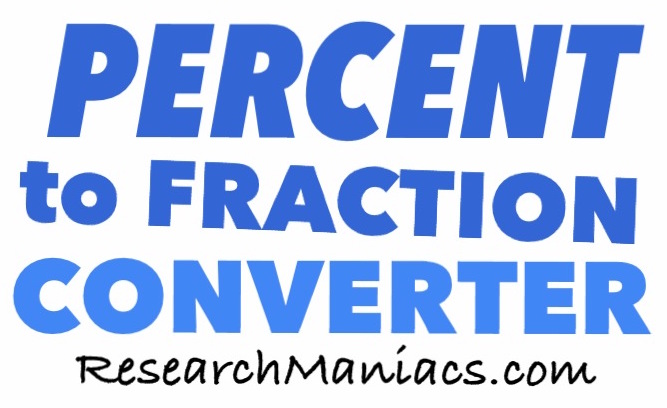Percent to Fraction ConverterWelcome to Research Maniacs' Percent to Fraction Converter.

To convert a percent to a fraction, please enter it in the box below and then press "Fraction".

.   %

Not sure what to convert? Try one of these:

What is 2.5% as a fraction?

What is 9.25% as a fraction?

What is 25.20% as a fraction?

What is 60% as a fraction?